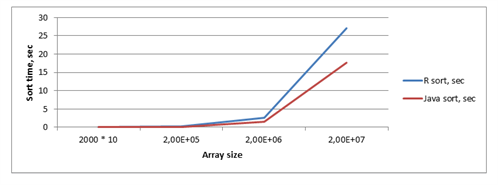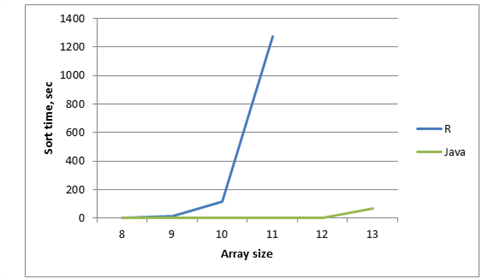## Topics

### Tags

A
B
C
D
E
F
G
I
L
M
N
P
R
S
T
W
Just start typing...Technologies

# R performance optimization using Java

Published April 21, 2015

Let us show how can a poor R performance be improved using rJava package. rJava provides a low-level bridge between R and Java (via JNI), and allows you to create objects, call methods and access fields of Java objects from R.R is a programming language and software environment for statistical computing and graphics. The R language is widely used among statisticians and data miners for developing statistical software and data analysis. R is freely available under the GNU General Public License, and pre-compiled binary versions are provided for various operating systems.

R has become the most popular language for data science and an essential tool for life science software development. R development, optimization, R algorithms implementation are successfully applied in WaveAccess science-intensive projects for the processing of large datasets.

However, if you have to work with big data you will face some performance issues because of :

1. Extreme dynamism

R is an extremely dynamic programming language, and almost anything (body, arguments and environment of functions, the S4 methods for a generic, add new fields to an S3 object, or even change its class, etc.) can be modified after it is created.

2. Name lookup with mutable environments

It's surprisingly difficult to find the value associated with a name in the R-language because of the combination of lexical scoping and extreme dynamism.

In R, functions' arguments are evaluated lazily. To implement a lazy evaluation, R uses a promise object that contains the expression needed to compute the result, and the environment in which to perform the computation. Creating these objects has some overhead, so that every additional argument to an R function slows it down a little.

Let me show how we can improve poor R performance using rJava package . rJava provides a low-level bridge between R and Java (via JNI). It allows you to create objects, call methods and access fields of Java objects from R.

##### Accessing java-methods from R
1. Assume that we have java class that provides us with the sorting of simple arrays of integers.

``````
import java.util.Arrays;
public class JavaSamples {

public static int[] sort(int[] array) {
Arrays.sort(array);
return array;
}
}
```
```
2. Let us compile our class source code with a javac command. You should see the new file (JavaSamples.class) in your working directory (for instance, "D:/R optimizations/"))

```        ```
> javac JavaSamples.java
```
```

First of all, you should check the environment variables' existence and correctness – java, javac, and R.

3. After starting R console, install (if it was not installed before) and load the rJava package.

```        ```
>> install.packages("rJava")
>> require(rJava)
```
```
4. Set the working directory to the selected folder (which contains the JavaSamples.class file).

```        ```
>>> setwd("D:/R optimizations/")
```
```
5. Initialize JVM in the working directory.

```        ```
>> .jinit('.')
```
```

If you need to attach an additional jars file, you should use the jaddClassPath command.

Now we have access to our java class JavaSamples. We can create a JavaSamples object, and call sort method with integer arrays as the first java method parameter. For converting the R vector to link to a java array we should use the .jarray method.

```        ```
>> javaObj <- .jnew("JavaSamples")
>> result <- .jcall(javaObj, "[I", "sort", .jarray(array))
```
```

Pay attention to the type defining of java returning value. In our case we should use "[I" definition (you can find a complete type description in table 1).

6. Result object – sorted with the java-method vector.

Table 1 - Overview of the abbreviations that can be used as a shortcut to indicate the return type of a Java method in the .jcall function of the rJava package (Java Native Interface).

 Abbreviation Type "V" void "I" integer "D" double (numeric) "J" long "F" float "Z" Boolean "C" char (integer) "S" string "B" byte (raw) "L" Java object of class (e.g. "Ljava/lang/Object" ) "[" Array of objects of type (e.g. "[D" for an array of doubles)
##### Java pack building

We have to add a few steps to the building package process for supporting our java sort method in the R package. Before detailing the contents of the individual files and folders, we provide a summary folder overview in Figure 1.

javaSortPack

- inst

- java

- javaSamples.jar

- java

- JavaSamples.java

- man

- JavaSamples.Rd

- R

- javaSamples.R

- DESCRIPTION

- NAMESPACE

Figure 1: Overview of the package contents for the javaSortPack package

1. We create the folder inst/java to host our JAR file. This JAR file contains a single JavaSamples.class file, generated from the following JavaSamples.java file. If we need to use other jars we should also add them to the inst/java folder.

```        ```
> jar cvf program.jar -C path/to/classes.
```
```

For building a jar archive, use jar command .

2. It is recommended to make Java source files available in a top-level java/ directory inside the package or in the jar itself.

3. Two functions are contained in the R/ subfolder of the package. The first function .onLoad is the function that will assure that the Java code is made available. The second function will be the R wrapper to execute the Java JavaSamples class. The .onLoad function is a hook function that will be run immediately after loading the package. The function .jpackage that is called inside the .onLoad function takes care to initialize the JVM and to add the java/ folder of the package to the class path.

```        ```
#options(java.parameters = "-Xmx2000m")
.jpackage(pkgname, lib.loc = libname)
}
```
```

For increasing the size of memory allocated for Java function we can use the options command.

4. The second function will be the R wrapper to execute the Java JavaSamples class. Function definition is the same as in the previous part of article.

```        ```
javaSort <- function(array) {
# creating object of java class JavaSamples
javaObj <- .jnew("JavaSamples")

# call java sort function, pass array as first java function's parameter
# returned object - array of integers
result <- .jcall(javaObj, "[I", "sort", .jarray(array)  )

return (result)
}
```
```
5. Build an R package in the regular way .

##### Testing

Array sorting

Let’s compare R and java performance on simple array sorting. Testing script:

```    ```
sort_iterations = 10

r_sort <- function(array) {
result <- sort(array)
return (result)
}

java_sort <- function(array) {
javaObj <- .jnew("JavaSamples")

result <- .jcall(javaObj, "[I", "sort",
.jarray(array)
)

return (result)
}

length = 2000 * 10
array <- sample.int(length, replace = TRUE)

print_time(for (i in 1:sort_iterations) { r_sort(array) })
print_time(for (i in 1:sort_iterations) { java_sort(array) })
```
```

Dependency of the function execution time and arrays size is shown in table 2 and on figure 2.

Table 2 - Dependency of function execution time and arrays size (sort iterations = 10)

 `Array size` `R sort, sec` `Java sort, sec` `2000 * 10` `0.02` `0.02` `2e+05` `0.17` `0.14` `2e+06` `2.67` `1.53` `2e+07` `27.05` `1761`Figure 2 – Chart with dependency of sort time, array size and method

##### Permutations

Let’s compare the time of two implementations of generating permutations in an array. You can find java and R code in the attachment below (table 3 and figure 3).

Table 3 - Dependency of the function execution time and array size

 `Array size` `8` `9` `10` `11` `12` `13` `R` `1.27` `11.41` `115.11` `1274.12` `Java` `0` `0` `0.04` `0.47` `4.97` `67.58`Figure 3 – Chart with dependency of execution time, array size and method

So, as you can see in both examples, using the java method in R is much more preferable in the case of a large amount of input data or using nonstandard data processing R algorithms. This method significantly increases algorithmic efficiency, reduces computations runtime and requires fewer system resources.

1. R performance discussion

http://stat.ethz.ch/education/semesters/ss2015/Progr_R3/Performance.Rmd

2. rJava package

https://www.rforge.net/rJava/

3. Command jar description

https://docs.oracle.com/javase/tutorial/deployment/jar/build.html

4. 4. Creating R extensions

http://cran.r-project.org/doc/manuals/r-release/R-exts.html

5. 5. Complete example of starting java functions in R

ftp://cran.r-project.org/pub/R/web/packages/helloJavaWorld/vignettes/helloJavaWorld.pdf

##### Attachment

JavaSamples.java

```    ```
import java.util.Arrays;

public class JavaSamples {

public static int[] sort(int[] array) {
Arrays.sort(array);
return array;
}

public static int javaPermutateArray(int[] array) {
return gen(array, 0, array.length);
}

private static int gen(int[] array, int cur, int length) {
int result = 0;

if (cur == length) {
result = 1;
} else {
for (int index = cur; index < length; ++index) {
swap(array, index, cur);
result += gen(array, cur + 1, length);
swap(array, index, cur);
}
}

return result;
}

private static void swap(int[] array, int i, int j) {
if (i != j) {
int tmp = array[i];
array[i] = array[j];
array[j] = tmp;
}
}
}
```
```
##### permutationArray.R
```    ```
# Path to this file
setwd('D:/R optimizations')

require(rJava)

.jinit('.')

swap <- function(array, i, j) {
if (i != j) {
tmp <- array[i]
array[i] <- array[j]
array[j] <- tmp
}

return (array)
}

gen <- function(array, cur, len) {
result <- 0

if (cur > len) {
result = 1;
} else {
for (index in cur:len) {
array <- swap(array, index, cur)
result <- result + gen(array, cur + 1, len)
array <- swap(array, index, cur)
}
}

return (result)
}

r_permutate_array <- function(array) {
result <- gen(array, 1, length(array))
return(result)
}

java_permutate_array <- function(array) {
javaObj <- .jnew("JavaSamples")

result <- .jcall(javaObj, "I", "javaPermutateArray",
.jarray(array))

return (result)
}

print_time <- function(x) {
print(system.time(x))
}

length = 7
array <- 1:length

print_time(r.result <- r_permutate_array(array))
print_time(java.result <- java_permutate_array(array))
```
```

## Related ServicesLife Sciences Software DevelopmentR Development & OptimizationReporting System DevelopmentHow we process your personal data

When you submit the completed form, your personal data will be processed by WaveAccess Services. Due to our international presence, your data may be transferred and processed outside the country where you reside or are located. You have the right to withdraw your consent at any time.